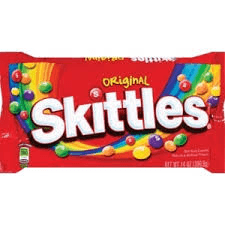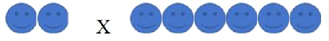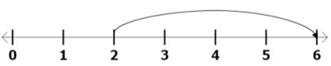Picture Models
Related Facts
Division
Word Problems
100

Identify the expression for this array:









3 x 4 =12

100

True or False:

Multiplication is a faster way to add?

What is...

True!

100

Name the related multiplication fact for this expression:

90/ 10 = 9

What is....

10 x 9

100

Solve:

3 ÷ 3=

What is...

1 or one

100Chris the alien has two hands. Each hand has four fingers on it. How many fingers does Chris have?

What is...

8 fingers or eight fingers

200

Identify the expression for this array:

xxxxx

xxxxx

5 x 2 = 10

200

True or False:

The expression below equals 30:

6+6+6+6+6+6

What is...

False.

The expression equals 36

200

Name the related multiplication fact for this
expression:

seven times five

5 x 7

200

Solve:

10 ÷ 2=

What is...

5 or five

200Leroy went to Walmart to buy a tricycle. Walmart only had three tricycles left. How many wheels did Leroy see?

What is...

nine wheels or 9 wheels

300

Draw an array to show 6 x 5 = 30

@@@@@@
@@@@@@
@@@@@@
@@@@@@
@@@@@@

300

What division equation is represented below?

50-5-5-5-5-5-5-5-5-5-5=0

What is...

50 ÷ 10 =5

300

Which fact below is related to the fact 9 x 10=90?

A) 9 x 5=45

B) 10 x 4=40

C) 90 ÷ 9=10

D) 2 x 1=2

What is...

C) 90 ÷  9=10

300

Solve:

21 ÷ 3=

What is...

7 or seven

300Christina bought six bags of skittles. Each bag has four skittles in it. How many skittles did Christina get?

24 skittles or twenty-four skittles

400

Choose two correct answer choices. Diego makes two different models that show 2 x 6. Which are Diego's models?

A)  6 + 6 = 12

B)C)D)What is...

A and B

400

Write a repeated addition number sentence for the following multiplication fact:

4 x 4=16

What is...

4+4+4+4=16

400

A) 5 x ? =15

B) 15 x 3=?

C) 3 + 5=?

D) 5 - 3 = ?

What is...

A) 5 x ? = 15

400

Solve:

Twenty divided by five equals

What is...

4 or four

400Andres bought fifty candy canes. He went to sleep at 2 o'clock. When he woke up he walked two miles. Andres has to share all his candy canes with five friends evenly. How many candy canes does each of his friends receive?

What is...

Ten candy canes or 10 candy canes

500

Draw an array to show:

1   x 5 = 5

%%%%%

500

Write a repeated subtraction number sentence for the following division fact:

40 ÷ 5=8

What is...

40-8-8-8-8-8=0

or

40-5-5-5-5-5-5-5-5=0

500

Which multiplication fact will fill in the blank to make this equation true? 45 = ___

A) 8 x 5

B) 3 x 6

C) 0 x 45

D) 9 x 5

What is...

D) 9 x 5

500

Solve:

20 ÷  4 =?

What is...

5 or five

500Sue, Steve, Sammy, Syndey, and Craig are all in the same class. Mrs. Pettigrew gives them each eight pencils. They have a class pet named Tom the Cat. How many pencils do the students have all together?

What is...

40 pencils or forty pencils

600

What is the expression for this array?

##
##
##
##
##
##

2 x 6 = 12

600

Write a repeated addition number sentence for the following multiplication problem:

10 x 10 =100

What is...

10+10+10+10+10+10+10+10+10+10=100

600

Name the multiplication fact that can help you solve this problem: 40 ÷ 5 =?

What is...

5 x 8

600

Solve:

36 ÷ 6=?

What is...

6 or six

600Cookie Monster went to the store and bought an enormous bag of OREOs. The bag has seventy OREOs. Cookie Monster eats seven cookies everyday. How many days would it take for him to eat all seventy cookies?

What is...

Ten Days or 10 Days

Click to zoom